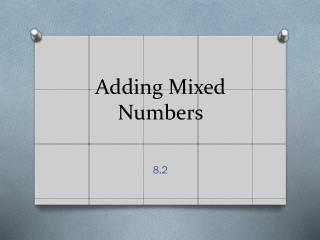DownloadDownload PresentationDownload Presentation- - - - - - - - - - - - - - - - - - - - - - - - - - - E N D - - - - - - - - - - - - - - - - - - - - - - - - - - -
##### Presentation Transcript

1. What would you do? 3 1/8 + 5 3/8

2. Procedure • Add the whole-number parts • Add the fraction parts • Rename into simplest form

3. Practice Renaming 4 7/5 8 4/3 2 7/4

4. Practice • MJ page 254 • Complete independently. • Stand behind your chair when you are finished.

5. Procedure for adding unlike denominators • Add the whole-number parts • Find a common denominator • Add the fraction parts • Rename into simplest form

6. Spiral Practice 6 7/8 2 ½ + 4 3/8= 5 2/3 + 1 5/6= 3 4/5 + 2 ¼= 6 5/6 + 3 3/5= 1 7/8 + 8 1/6= 7 1/2 6 1/20 10 13/30 10 1/24

7. Pattern • Look at the following problems and determine a pattern: 1 + 1 = 3 1 2 6 1 + 1 = 5 2 3 6 1 + 1 = 7 3 4 12 1 + 1 = 9 4 5 20

8. Solution • When you are adding two unit fractions (numerators are 1), the sum will always be the sum of the denominators product of the denominators (How cool is that!?!)

9. Pattern Take a look again to see the pattern: the sum of the denominators product of the denominators 1 + 1 = 3 1 2 6 1 + 1 = 5 2 3 6 1 + 1 = 7 3 4 12 1 + 1 = 9 4 5 20

10. Practice the Pattern • Use the pattern on these fractions – remember this only works with unit fractions 1/3 + 1/5 = 1/12 + 1/ 4 = 1/9 + 1/7 = 1/5 + 1/15 =

11. Subtracting Mixed Numbers(notes) • Subtract the fractions first in case you need to borrow from the whole number. • Be sure you have common denominators before subtracting • Subtract the whole numbers. Ta Da you are a genius!

12. Let’s Practice Borrowing • 7 ¼ - 5 3/5= • 6 1/2 - 3 2/3= • 9 1/3 - 5 2/4= • 10 1/5 - 3 ½= 1 13/20 2 5/6 3 5/6 6 7/10

13. What about these? • 6 - ¼ = • 8 – 3 2/3= • 4 3/5- 1 4/5= • 5 1/6 – 2 5/6= 5 3/4 4 1/3 2 4/5 2 1/2

14. Mixed Number Spin • Make a spinner from a paper clip and a pencil point • Use the mixed numbers from your spin to create true number sentences • The first person to create 10 true sentences is the winner